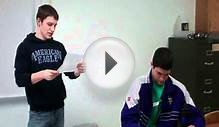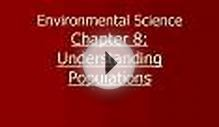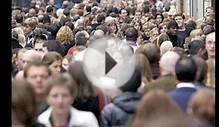# Environmental Science Population

May 11, 2022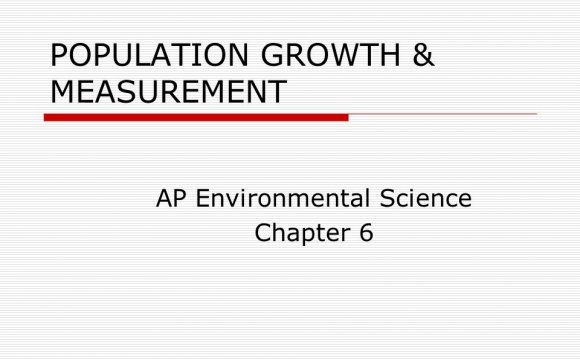Environmental scientists use two models to describe how populations grow over time: the exponential growth model and the logistic growth model. Two important concepts underlie both models of population growth:

• Carrying capacity: Carrying capacity is the number of individuals that the available resources of an environment can successfully support. In equations and models, the symbol represents carrying capacity.
• Limiting resource: A limiting resource is a resource that organisms must have in order to survive and that is available only in limited quantity in their environment. Therefore, a limiting resource functions to limit population growth. Food and water are common limiting resources for animals.

### Exponential population growth model

In the exponential growth model, population increase over time is a result of the number of individuals available to reproduce without regard to resource limits. In exponential growth, the population size increases at an exponential rate over time, continuing upward as shown in this figure.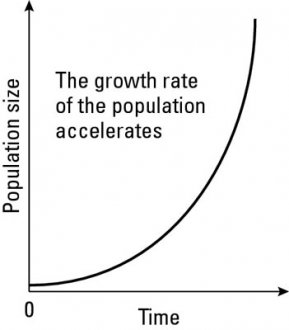The line, or curve, you see in the figure shows how quickly a population can grow when it doesn’t face any limiting resources. The line creates a shape like the letter and is sometimes called a J-curve.

Scientists often describe models with equations. The exponential growth model equation looks like this:

dN/dt = rN

The symbols in this equation represent concepts. Here’s how to translate the equation into words: The change (d) in number of individuals (N) over a change (d) in time (t) equals the rate of increase (r) in number of individuals (N).

### Logistic population growth model

In reality, the growth of most populations depends at least in part on the available resources in their environments. To model more realistic population growth, scientists developed the logitic growth model, which illustrates how a population may increase exponentially until it reaches the carrying capacity of its environment. When a population’s number reaches the carrying capacity, population growth slows down or stops altogether. This figure illustrates the logistic growth model.

In the logistic growth model, population size levels off because the limiting resources restrain any further growth. This model applies in particular to populations that respond to density-dependent factors. As you can see in the figure, the logistic growth model looks like the letter S, which is why it’s often called an S-curve.

Scientists describe the logistic growth model with the following equation, which uses the same symbols as the exponential growth model (see the preceding section):

dN/dt = rN (1 – N/K)

This equation says that the change (d) in number of individuals (N) over a change (d) in time (t) equals the rate of increase (r) in number of individuals where population size (N) is a proportion of the carrying capacity (K).

The best part about this equation is that it includes a way to factor in the negative feedback effect of a larger population relying on the same resources as a smaller population.

While the logistic growth model is often more descriptive of what occurs in reality than the exponential growth model, it still doesn’t accurately describe what usually occurs in real life. What scientists have actually observed in nature is that populations seldom reach the carrying capacity and remain stable. Rather, they experience a pattern called overshoot and die off.

As populations approach their carrying capacity, more offspring are born than the current resources can support; as a result, the population exceeds, or overshoots, the carrying capacity. When the population numbers exceed what the environment can support, some individuals suffer and die off because of the insufficient resources.

This figure shows what the pattern of overshoot and die off looks like. A common situation that leads to this pattern is the variation in resource availability from year to year. For example, although plenty of food is available this spring while a population is reproducing, by the time the offspring are born, the food resources may have shifted enough that they can’t support all the new offspring.